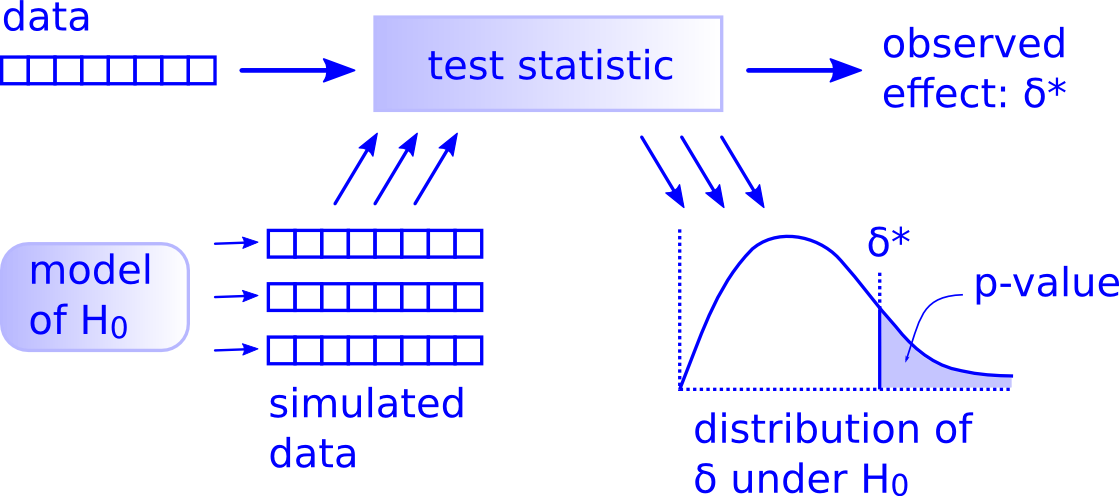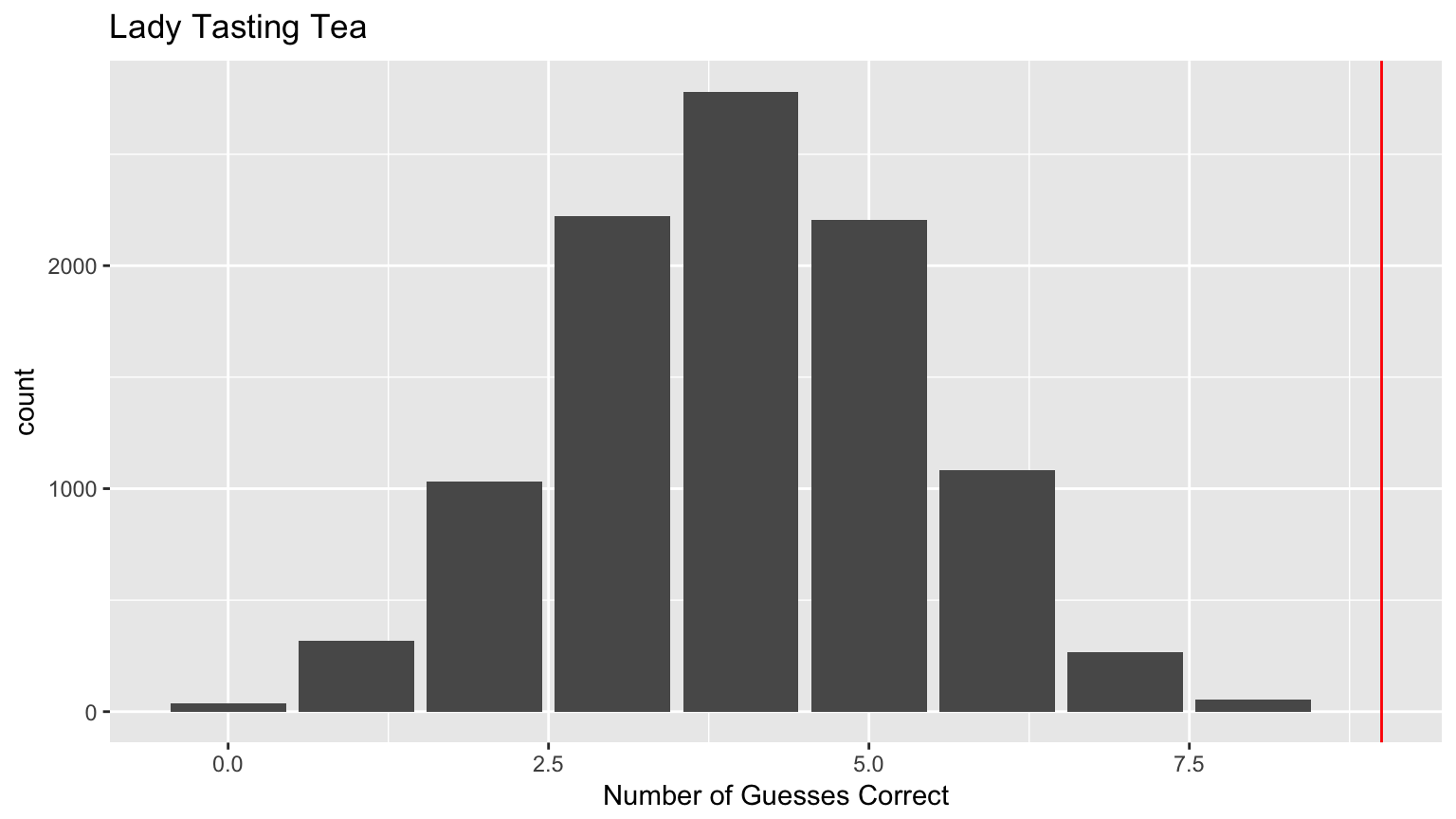## The process for creating a confidence interval

#### From Monday

• Obtain an original sample (at random) & compute the original sample statistic
• Lay out what the null and alternative hypotheses are
• Determine what sort of random process assumes $$H_0$$ while keeping true to how the original sample was selected and the original statistic was calculated
• Create the randomization distribution by repeating the process many times
• Shift this distribution by adding the original statistic (point estimate)
• Compute the appropriate percentiles from this shifted distribution
• 2.5 and 97.5 for 95% confidence level
• 5 and 95 for 90% confidence level
• Interpret the resulting confidence interval in the context of the problem

## The process for creating a confidence interval

#### Using bootstrapping

• Obtain an original sample (at random) & compute the original sample statistic
• Bootstrap the original sample (sample with replacement of the same size as the original sample) in a way similar to how the original sample was collected
• You may need to group your data as needed and then compute the bootstrap statistic from your bootstrap sample
• Create the bootstrap distribution by repeating the process (in the last two steps) many times
• Compute the appropriate percentiles from this bootstrap distribution
• 2.5 and 97.5 for 95% confidence level
• 5 and 95 for 90% confidence level
• Interpret the resulting confidence interval in the context of the problem

## Wrap Up

1. In general: What 3 components exist for all hypothesis tests
2. Specifically: How the 3 components vary by setting

## There is Only One TestImage source here

## Hypothesis Testing in General

The how of these 3 points may change, but the what doesn't:

1. Identify the test statistic
2. Construct the null distribution of the test statistic based on $$H_0$$
3. Compare the observed test statistic to the null distribution to compute the p-value

## Specifics 1: Test Statistic

We can do hypothesis testing for different scenarios

Type Population Parameter Test Statistic
One-Sample Mean $$\mu$$ Sample Mean $$\overline{x}$$
One-Sample Proportion $$\pi$$ Sample Proportion $$\widehat{p}$$
Two-Sample (Independent) Diff of Means $$\mu_1 - \mu_2$$ $$\overline{x}_1 - \overline{x}_2$$
Two-Sample (Paired) Mean Difference $$\mu_{diff}$$ $$\overline{x}_{diff}$$
Two-Sample Diff of Proportions $$\pi_1 - \pi_2$$ $$\widehat{p}_1 - \widehat{p}_2$$

## Specifics 2: Null Distribution

We can construct the null distribution of the test statistic either

• Via computation i.e. simulation/shuffling
• Ex: We used the Permutation Test i.e. we permuted things
• Via mathematics i.e. analytically
• Ex: Traditionally via Probability using the Central Limit Theorem: $$\overline{X}$$ is Normally distributed as $$n \rightarrow \infty$$
• Note: the null distribution isn't always bell shaped! i.e. not always Normal

## Specifics 3: p-Value

Depending on the alternative hypothesis $$H_A$$, we have either

1. Two-sided p-values. Ex:
• $$H_A: \mu_{1} - \mu_{2} \neq 0$$
2. One-sided p-values. Ex:
• $$H_A: \mu_{1} - \mu_{2} < 0$$
• $$H_A: \mu_{1} - \mu_{2} > 0$$

## Moral of the Story

If you forget what hypothesis testing and/or p-values are remember:## Split into your Final Project groups

For each of the following five problems, it may be helpful to identify

• the variables of interest,
• the types of variables they are,
• and the observational units

BEFORE trying to identify which type of problem it is.

AFTER identifying the problem type for each of the five problems, start to layout what the null and alternative hypotheses are in symbols.

## Identify problem types

Identify which of the following types of problems each of these corresponds to:

Types: One Mean, One Proportion, Two Means (Independent), Two Means (Paired), Two Proportions

Problem 1: Average income varies from one region of the country to another, and it often reflects both lifestyles and regional living expenses. Suppose a new graduate is considering a job in two locations, Cleveland, OH and Sacramento, CA, and he wants to see whether the average income in one of these cities is higher than the other. He would like to conduct a hypothesis test based on two randomly selected samples from the 2000 Census.

## Identify problem types

Identify which of the following types of problems each of these corresponds to:

Types: One Mean, One Proportion, Two Means (Independent), Two Means (Paired), Two Proportions

Problem 2: A 2010 survey asked 827 randomly sampled registered voters in California "Do you support? Or do you oppose? Drilling for oil and natural gas off the Coast of California? Or do you not know enough to say?" Conduct a hypothesis test to determine if the data provide strong evidence that the proportion of college graduates who do not have an opinion on this issue is different than that of non-college graduates.

## Identify problem types

Identify which of the following types of problems each of these corresponds to:

Types: One Mean, One Proportion, Two Means (Independent), Two Means (Paired), Two Proportions

Problem 3: The CEO of a large electric utility claims that 80 percent of his 1,000,000 customers are satisfied with the service they receive. To test this claim, the local newspaper surveyed 100 customers, using simple random sampling. 73 were satisfied and the remaining were unsatisfied. Based on these findings from the sample, can we reject the CEO's hypothesis that 80% of the customers are satisfied?

## Identify problem types

Identify which of the following types of problems each of these corresponds to:

Types: One Mean, One Proportion, Two Means (Independent), Two Means (Paired), Two Proportions

Problem 4: The National Survey of Family Growth conducted by the Centers for Disease Control gathers information on family life, marriage and divorce, pregnancy, infertility, use of contraception, and men's and women's health. One of the variables collected on this survey is the age at first marriage. 5,534 randomly sampled US women between 2006 and 2010 completed the survey. The women sampled here had been married at least once. Do we have evidence that the mean age of first marriage for all US women from 2006 to 2010 is greater than 23 years?

## Identify problem types

Identify which of the following types of problems each of these corresponds to:

Types: One Mean, One Proportion, Two Means (Independent), Two Means (Paired), Two Proportions

Problem 5: Trace metals in drinking water affect the flavor and an unusually high concentration can pose a health hazard. Ten pairs of data were taken measuring zinc concentration in bottom water and surface water at 10 randomly selected locations on a stretch of river. Do the data suggest that the true average concentration in the surface water is smaller than that of bottom water? (Note that units are not given.)

• Work on replicating the code in your own R Markdown file from these worked out examples on the Coggle Mind Map here
• This should be good practice for when you do your final project on how I'd like you to perform your inferential statistics
• After reviewing the resampling/bootstrapping, look over how inference is traditionally taught using formulas
• I'll be walking around the room and asking you what the pieces of code in the examples do
• Here are the problem assignments by group:
• Griffin, Jenny, Dinisa, Kawita: Two Means (Paired)
• Cassie, Hunter, Emily, Liz: Two Proportions
• Wyatt, Nick, Xavier, Gray: Two Means (Independent)
• Max, Nani, Daniel, Kelcie: One Mean
• Meaghan, Rachel, Brittany, Kyle: One Proportion

## Walk through paired example

• Let's take notes on the printed out "There is Only One Test" diagram
• The direct link to the example is here

## To do for next time

• Don't just produce the basic ggplot plots. Jazz them up!